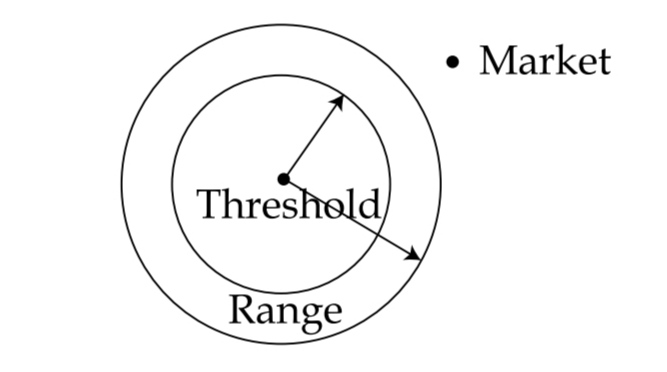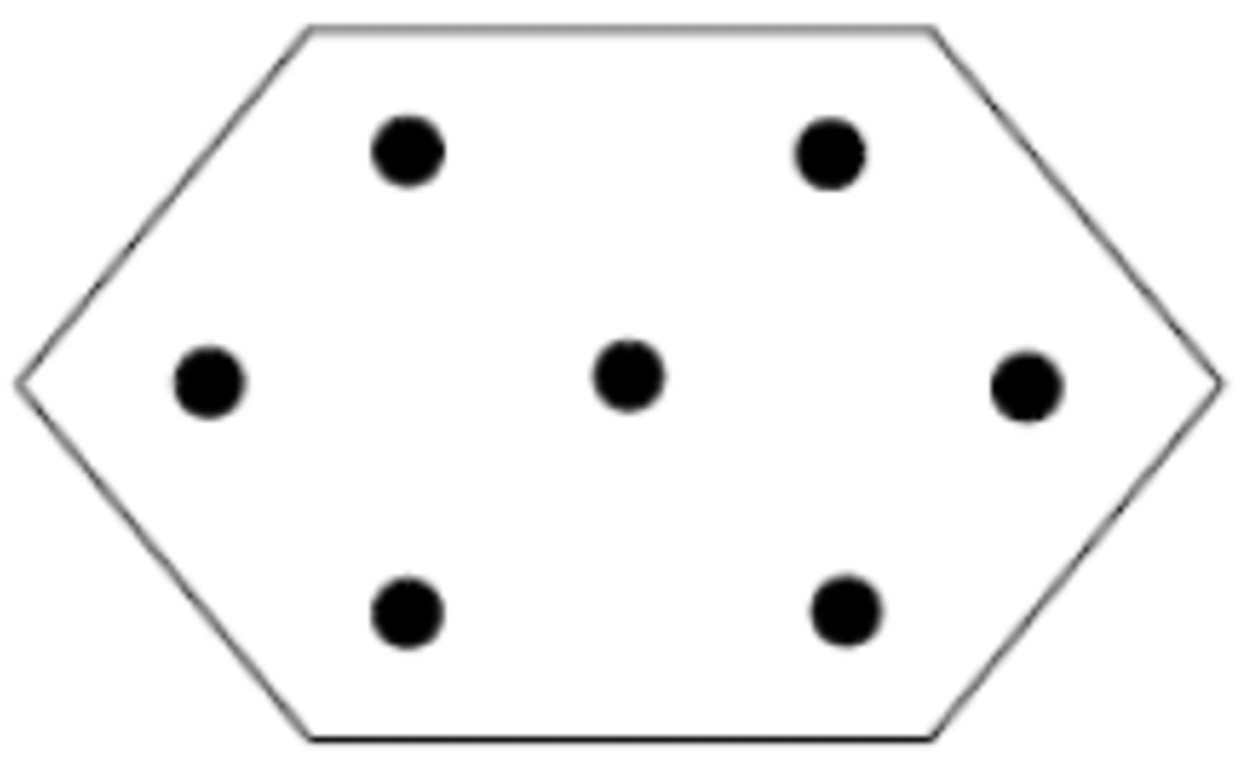# SETTLEMENT GEOGRAPHY PYQS JULY & DEC 2018 NTA UGC NET

SETTLEMENT GEOGRAPHY PYQS JULY & DEC 2018 NTA UGC NET

SETTLEMENT GEOGRAPHY PYQS 2018 NTA UGC NET, SETTLEMENT GEOGRAPHY  NTA UGC NET NOV 2018,  SETTLEMENT GEOGRAPHY PYQS UGC NET JULY 2018, SETTLEMENT GEOGRAPHY PYQS 2018, NTA UGC NET PYQS GEOGRAPHY, SETTLEMENT GEOGRAPHY PYQS UGC NET, GEOGRAPHY PYQS UGC NET,

(PDF) For download PDF file of JULY & DEC 2018 UGC NET – go to the bottom of this post.

Q.1. Which one of the following statements correctly depicts the threshold according to Central Place Model? (UGC NET JULY 2018)

(1) Point at which consumer movement is minimum

(2) Distance far which consumer will travel for a service

(3) Minimum number of people needed to support a service

(4) Economic base of a centre

Q.2. Which one of the following Models is explained by the given figure below? (UGC NET JULY 2018)(1) Weber’s Model

(2) Central Place Model

(3) Von Thunen Model

(4) Gravity Model

Q.3. Match the List-I with List – II and select the correct answer from the code given below: (UGC NET JULY 2018)

List – I (Settlement Alignment)List – II (Settlement Pattern)

(i) Uniform

(ii) Star Shaped

(iii) Linear

(iv) Cluster

Code:

(a)  (b)  (c)  (d)

(1)  ii     iii   iv    i

(2) iii    iv     ii    i

(3) i     ii     iii     iv

(4)  iv   iii    ii      i

Q.4. Given below are two statements, one is labelled as Assertion (A) and the other is labelled as Reason (R). Read the statements and choose the correct answer using the code given below. (UGC NET DEC 2018)

Assertion (A): Central Business District (CBD) of a city has high concentration of wholesale stores, offices and cultural and recreational activities.

Reason (R): Prices and demand of real estate increases as distance towards CBD reduces.

Code:

(1). (A) is true, but (R) is false.

(2). Both (A) and (R) are true, but (R) is not the correct explanation of (A)

(3). Both (A) and (R) are true and (R) is the correct explanation of (A)

(4). (A) is false, but (R) is true.

Q.5. Given below are two statements, one is labelled as Assertion (A) and the other is labelled as Reason (R. Read the statements and choose the correct answer using the code given below (UGC NET DEC 2018)

Assertion (A): The decade of 1990s witnessed urbanisation process speeding up economic growth in India.

Reason (R): The urbanisation process resulted in a growing shortage of urban services and an overall deterioration in the quality-of-urban-life indices.

Code:

(1) (A) is false, but (R) is true

(2). Both (A) and (R) are true and (R) is the correct explanation of (A)

(3). Both (A) and (R) are true, but (R) is not the correct explanation of (A)

(4). (A) is true, but (R) is false

Q.6. Match List I with List II and choose the correct answer from the code given below (UGC NET DEC 2018)

List I

(Model Concept)

(a) Sector Model

(b) Concentric Zone Model

(c) Bid-Rent theory

List II

(Definition)

(i) Model of the internal structure of cities in which social groups are spatially arranged in a series of rings

(ii) Nucleus of a city where retail stores and offices are concentrated

(iii) Theory that refers how price and demand on real estate changes as distance towards CBD increases

(iv) Model of internal structure of cities in which social groups are arranged around a series of sectors or wedges radiating from CBD

Code:

(1). (a)-(ii), (b)-(i), (c)-(iv), (d)-(iii)

(2). (a)-(iv), (b)-(i), (c)-(iii), (d)-(ii)

(3). (a)-(iii), (b)-(i), (c)-(iv), (d)-(ii)

(4). (a)-(i), (b)-(i), (c)-iv), (d)-(iii)

Q.7. Which one of the following principles is representative of the following figure of Christaller’s Model? (UGC NET DEC 2018)(1) Transport Principle

(3) Marketing Principle

(4) Threshold Principle

Q.8. Which one of the following economic principles is correct for organisation of settlements based on K= 4 presented in the Christaller’s central place model? (UGC NET DEC 2018)

(1) Agricultural activities

(2) Transport